# Chapter 4 Multivariate Visualization

library(tidyverse)
library(ggformula)
library(statthink)

theme_set(theme_statthinking(base_size = 14))

Real world data are never as simple as exploring a distribution of a single variable, particularly when trying to understand individual variation. In most cases attributes interact with one another, move in tandem, and many phenomena help to explain the attribute of interest. For example, when thinking about admission rates of higher education institutions, what may be some important attributes that would explain some of the reasons why higher education institutions differ in their admission rates? When thinking about the high temperature for the given day, what attributes would be helpful to understand why the high temperature on a day is different? Take a few minutes to brainstorm some ideas.

Building off of the high temperature example, we will use weather data from two seasons of cooler months of the year, October through April of 2018-2019 and 2019-2020, of various locations around the United States. This data was downloaded from the National Centers for Environmental Information (NCEI) Climate Data Online portal and is part of the companion package, statthink. The locations extracted in these data are found in the northern part of the United States and include: c(“Buffalo, NY”, “Iowa City, IA”, “Chicago, IL”, “Boston, MA”, “Portland, ME”, “Minneapolis, MN”, “Duluth, MN”, “Detroit, MI”). The first few rows of the data are shown below.

DT::datatable(us_weather)

## 4.1 Multivariate Distributions

Before moving to multivariate distributions, first, we will explore a univariate distribution of the average daily temperature. The average daily temperature takes the recorded temperatures from a single day and averages those, therefore this value would fall somewhere between the high and low temperature for the day. What are some key characteristics of the average daily temperature distribution? Take a few minutes to summarize the key characteristics.

gf_histogram(~ drybulbtemp_avg, data = us_weather, bins = 30) %>%
gf_labs(x = "Average daily temperature, in Fahrenheit",
title = "Univariate distribution of avg daily temperature")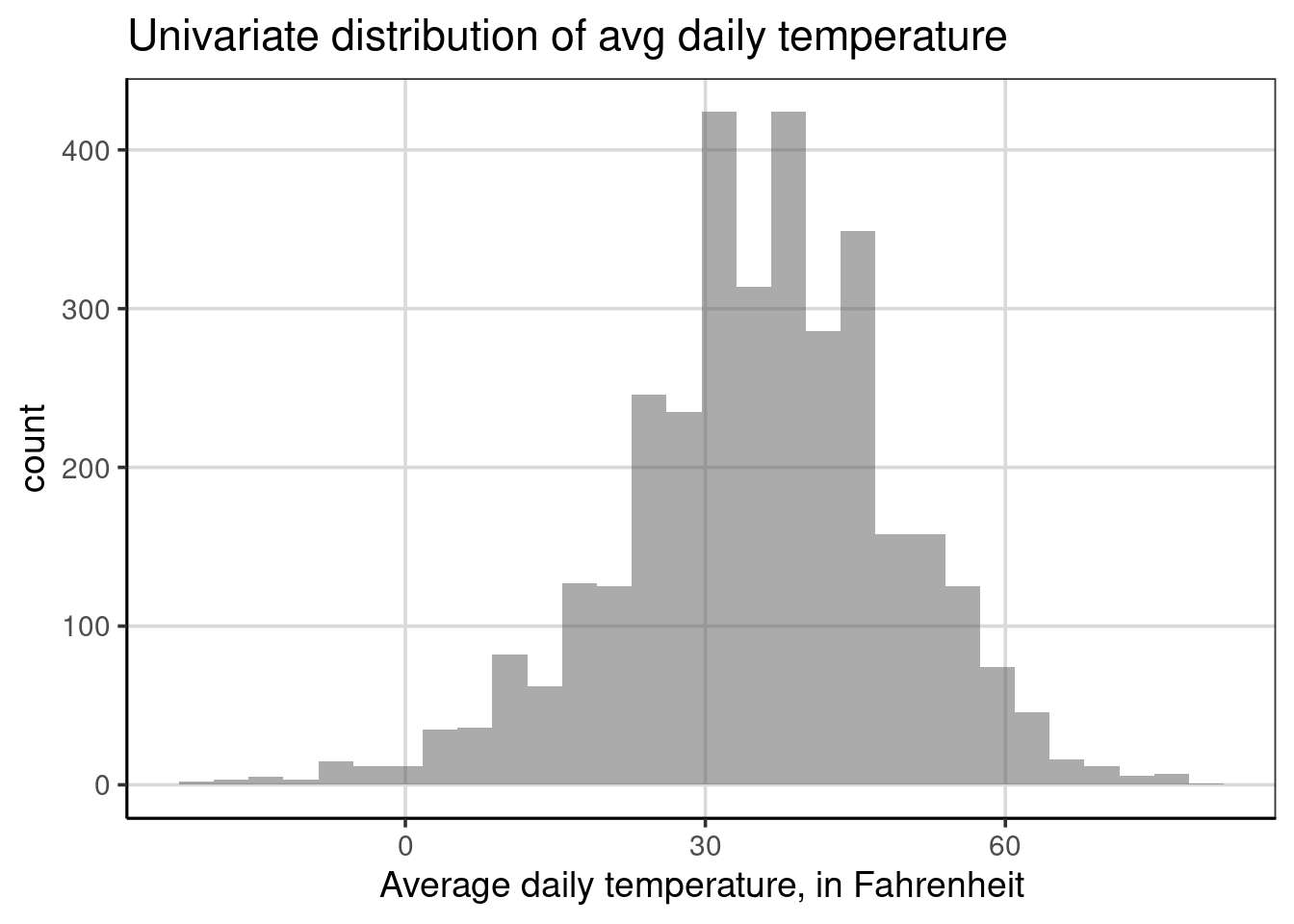From the univariate figure of the average daily temperature, you may have noticed that there is variation in these values, with a fairly wide range considering the values. For example, there are some average daily temperatures that are below zero and some of 60 degree Fahrenheit. However, most values are between 20 and 45 or so, which is reasonable given these are cooler months of the year and mostly northern location in the United States. Finally, the distribution is unimodal and somewhat symmetric, at least not skewed enough to be too concerned.

Earlier the question was asked what other attributes may help to explain why there are variations in high temperatures. If you did not think about that question then, we encourage you to think about this question now. What attributes could help to understand why there is such a wide range of values in the average daily temperature?

There are many answers that could be informative to this discussion, the one we will explore first is whether it snowed on a given day. This could influence the average temperature for a few reasons, first when it snows it must be cold enough for the precipitation to stay in frozen form rather than melting as it falls being at or below freezing (32 degrees Fahrenheit);16 also, if it snowed during the day it would also be less likely for the sun to be out further making the temperature less variable and likely lower during the winter months.

### 4.1.1 Histograms

Below is the multivariate distribution of the average daily temperature by whether it snowed or not at some point during that day. Whether it snowed or not is depicted by color in the figure, the blue color is showing the distribution of average daily temperature for days where it snowed and red is otherwise. Before we interpret the figure in more detail, the code for making this change was done by adding the snow attribute to the fill aesthetic using the formula syntax: fill = ~ snow. The fill aesthetic is telling the histogram bars to be colored by the different categories of the attribute of interest, here snow. The fill attribute for histrograms is best used when the attribute only has a few categories.

gf_histogram(~ drybulbtemp_avg, data = us_weather, bins = 30,
fill = ~ snow) %>%
gf_labs(x = "Average daily temperature, in Fahrenheit",
title = "Multivariate distribution of avg daily temperature by whether it snowed",
fill = "Snow?")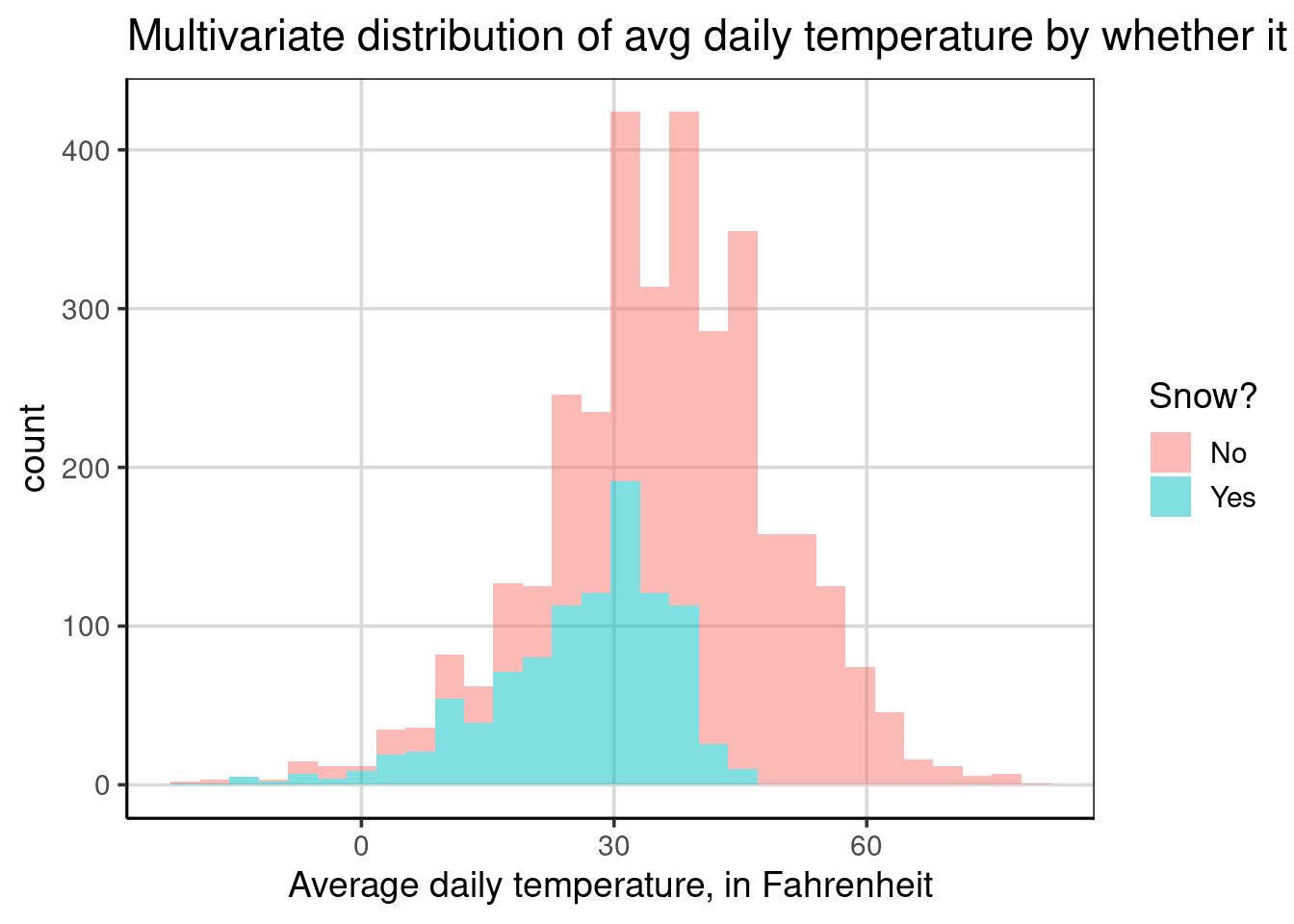If you were to compare the distribution for the average daily temperature for days when it snowed compared to when it did not snow, what similarities and differences do you notice? Take a few minutes to try and interpret this figure, focusing on characteristics that are similar or different across days when it snowed or not.

You may notice first that the bars are higher for the days that did not snow compared to days that it did snow. Why might this be occurring? If you said the reason is that it tends to snow less frequently, you would be correct. The bars are higher for days when it did not snow due to more data (i.e., days) where it did not snow. This characteristic of the histogram in this example makes the histogram more difficult to interpret and can even mislead when making comparisons across the two groups. We will explore a solution to this problem soon.

Another observation you may have noticed is that it appears the temperature tends to be lower for days in which it snows compared to those that it did not snow. On average, if you estimated the location of the mean for the two groups, the average daily temperature for days where it snowed would likely be just under 30 degrees where as it would be around 45 degrees Fahrenheit for days it did not snow. You may have also noticed that the variability for days when it snowed was also lower compared to days where it did snow. Why might this occur? This is likely due to the temperature ranges where snow forms readily.

You may wonder, why are there days where the average daily temperature was about 45 degrees Fahrenheit when it generally needs to be lower than 32 degrees Fahrenheit for it to snow? There could be many explanations for this, but since the distribution is showing the average daily temperature over a 24 hour period, the weather can change quite drastically over this period. For example, it could snow at the beginning or end of the day and the rest of the day could be quite different and much warmer than when it snowed.

Finally, you may also notice that the distribution for days when it snowed is somewhat more left skewed compared to days in which it does not snow. As snow readily forms when it is cooler, typically less than 32 degrees Fahrenheit, the temperature would commonly fall below this value when it snows. Therefore, the average temperature would be restricted by those colder values, pulling the average lower, even if it was warmer earlier or later in the day. This restriction is not found for days when it does not snow, therefore the temperatures are able to be found over a wider range of temperature values.

### 4.1.2 Density Curves

Often density plots are easier to visualize when there are more than one group. The other benefit of moving to a density plot is that any sample size differences across the groups are normalized automatically, which does not occur by default with the histogram. To start, we will explore the average daily temperature based on whether it snowed that day or not. For the first density curve, we will use almost the same code as the multivariate histogram. The only change in the code is to use gf_density() instead of gf_histogram(). Take a few minutes to summarize important differences in this figure compared to the multivariate histogram.

gf_density(~ drybulbtemp_avg, data = us_weather, size = 1,
fill = ~ snow) %>%
gf_labs(x = "Average daily temperature, in Fahrenheit",
title = "Multivariate distribution of avg daily temperature by whether it snowed",
fill = "Snow?")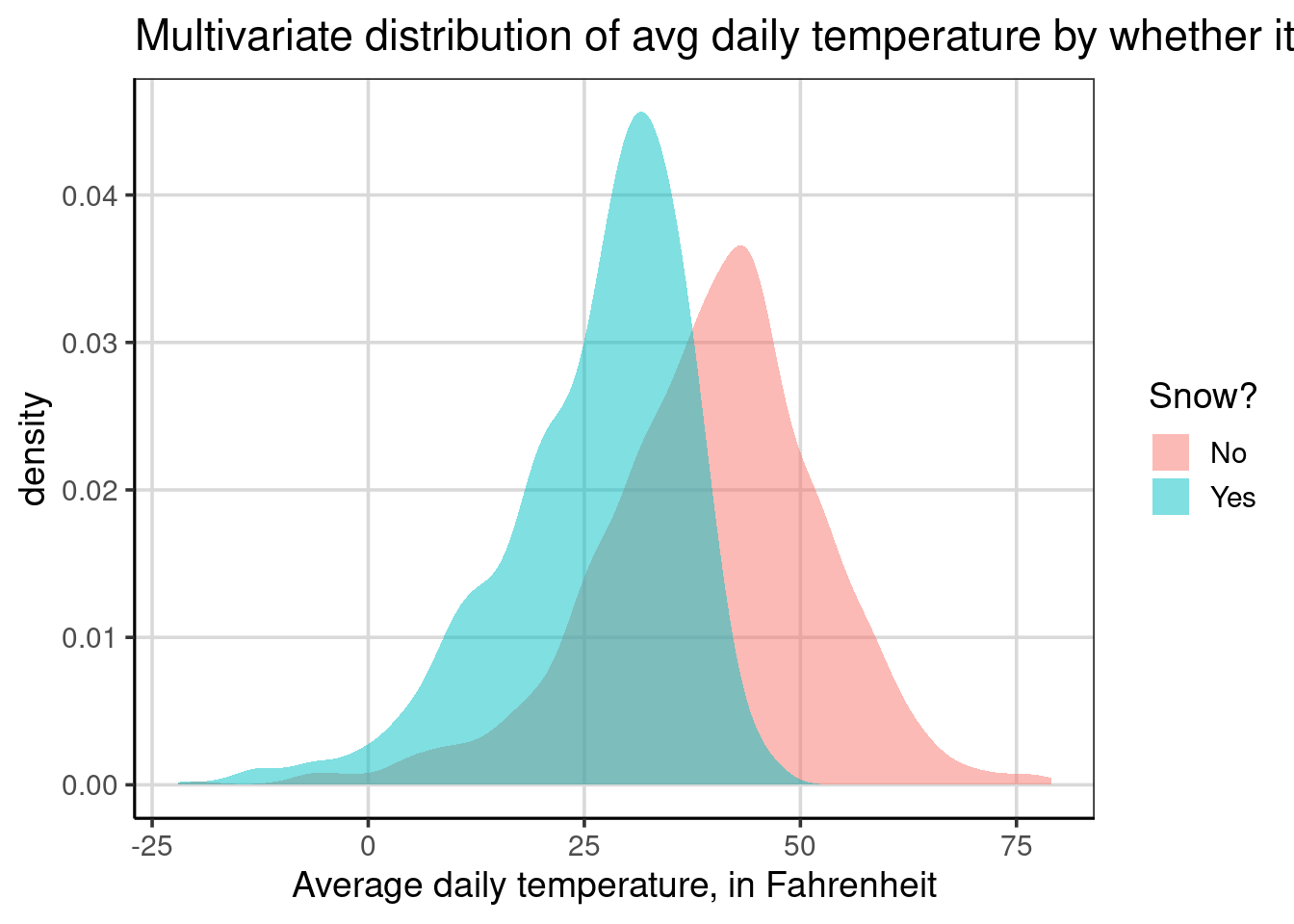Notice that there are two density curves, each shaded a different color, light blue for days in which it snowed, and light red for days where it did not snow. One primary difference, between the density and histogram is that the curves are normalized for sample size differences, therefore differences in heights for the two density curves can be interpreted as there being more data at that location. For example, the curve for days it snowed has a higher peak, around 30 degrees Fahrenheit, compared to the peak for days it did not snow, around 45 degrees. The higher peak here means there is more data clustered around 30 degrees for the snowy days compared to the amount of data clustered around 45 degrees for the days it did not snow.

The normalization of sample size across the groups helps to accentuate the key differences. Namely, there are differences in where the center and amount of variation between the two groups. When plotting two groups with a histogram, the groups are plotted over top of each other which can further mask differences in the group that is plotted first. The density curve with some transparency, as depicted above, does not suffer from these problems.

There is another plotting aesthetic that is useful to know about when using density curves. This is the color aesthetic which changes the colors of the line of the density curve. Similar to the fill aesthetic, it is possible to have the lines change color based on the snow attribute as such: color = ~ snow. The code below does this and also makes the lines slightly larger to view easier with the size = 1 global aesthetic.

gf_density(~ drybulbtemp_avg, data = us_weather,
color = ~ snow, size = 1) %>%
gf_labs(x = "Average daily temperature, in Fahrenheit",
title = "Multivariate distribution of avg daily temperature by whether it snowed",
color = "Snow?")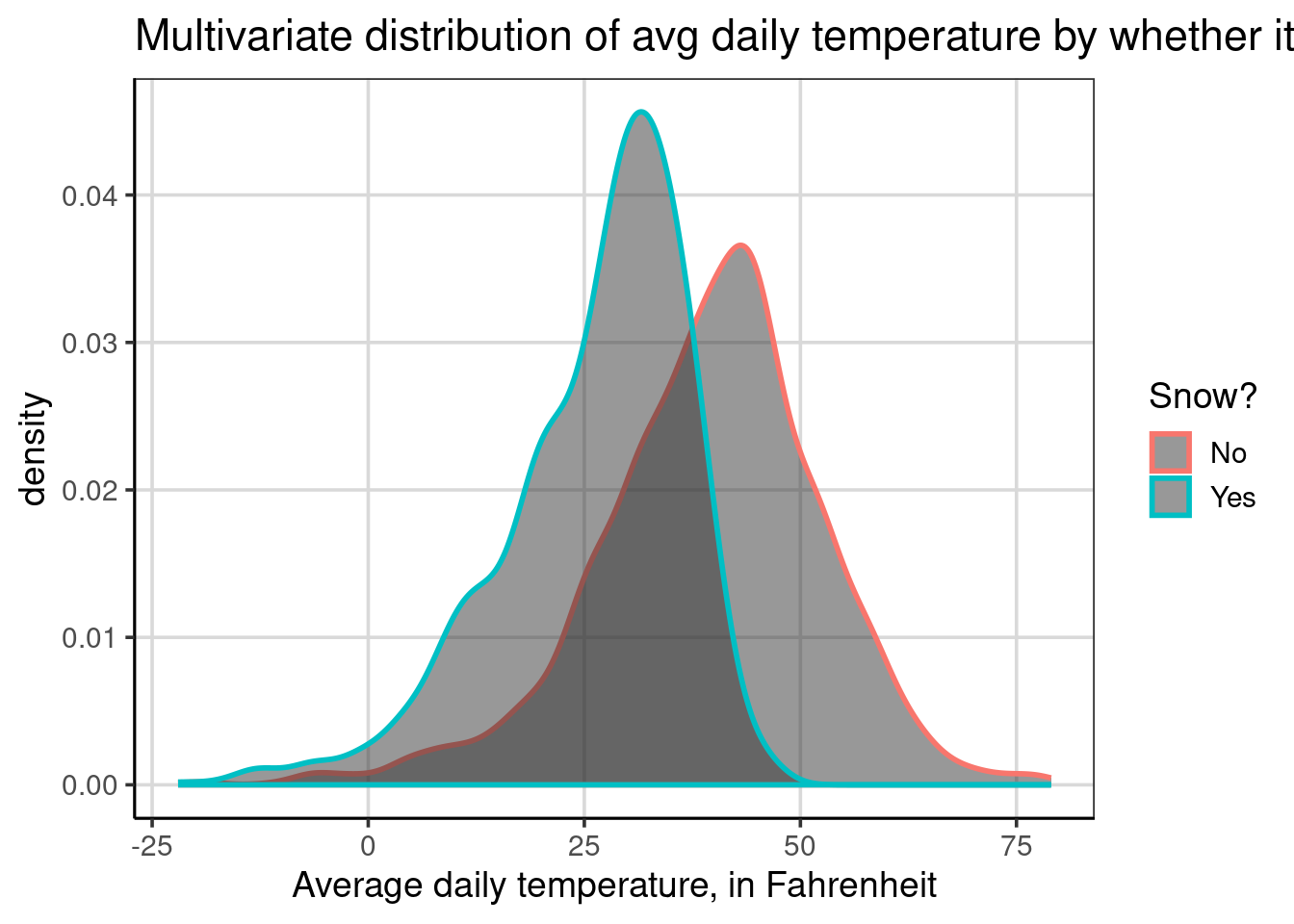Without specifying the fill aesthetic, both groups have the same fill color below the lines, but notice that now the lines are colored instead of the entire curve being filled in. Areas that are darker gray are areas where the two groups overlap. The rest of the density curves are the same besides the appearance differences.

It is also possible to combine the color and fill aesthetics, even with the same attribute. For example, the code below using the snow attribute for both the color and fill aesthetics. The primary difference here is that the lines are a bit larger compared to the first density figure shown.

gf_density(~ drybulbtemp_avg, data = us_weather,
color = ~ snow, size = 1,
fill = ~ snow) %>%
gf_labs(x = "Average daily temperature, in Fahrenheit",
title = "Multivariate distribution of avg daily temperature by whether it snowed",
fill = "Snow?",
color = "Snow?")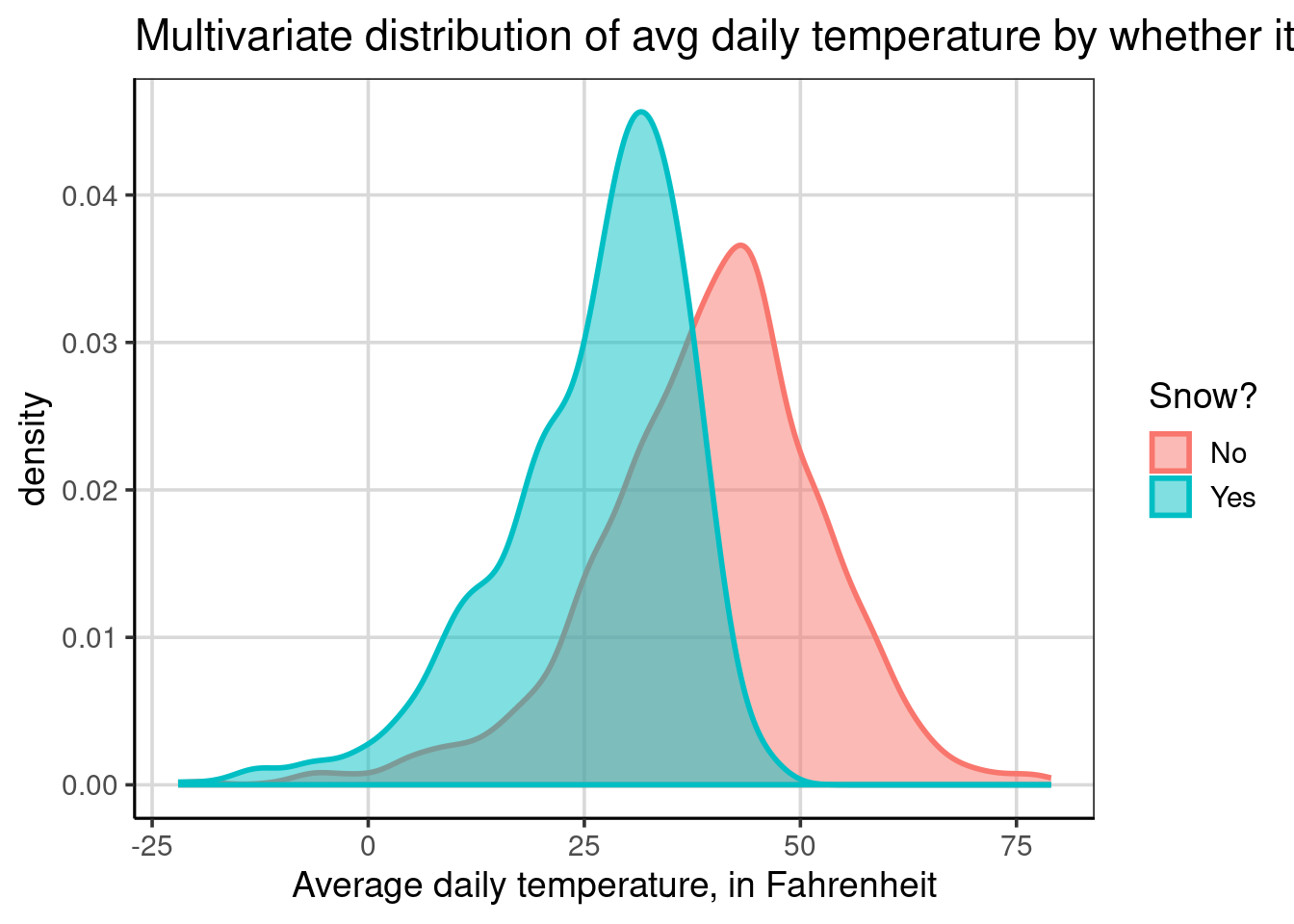It is also possible to use both aesthetics, but set one to be constant rather than adding the attribute to it. When there are many groups, this can be a good way to visualize more groups and not have too much color happening. In the code below, the snow attribute is specified to the color aesthetic, but now the fill aesthetic is set to a specific color, this time a shade of gray. The grayscale ranges from 0 to 100, where 0 is black and 100 is white, therefore in this case when setting fill = 'gray75' this would be a lighter gray as the number is closer to 100.

gf_density(~ drybulbtemp_avg, data = us_weather,
color = ~ snow, size = 1,
fill = 'gray75') %>%
gf_labs(x = "Average daily temperature, in Fahrenheit",
title = "Multivariate distribution of avg daily temperature by whether it snowed",
color = "Snow?")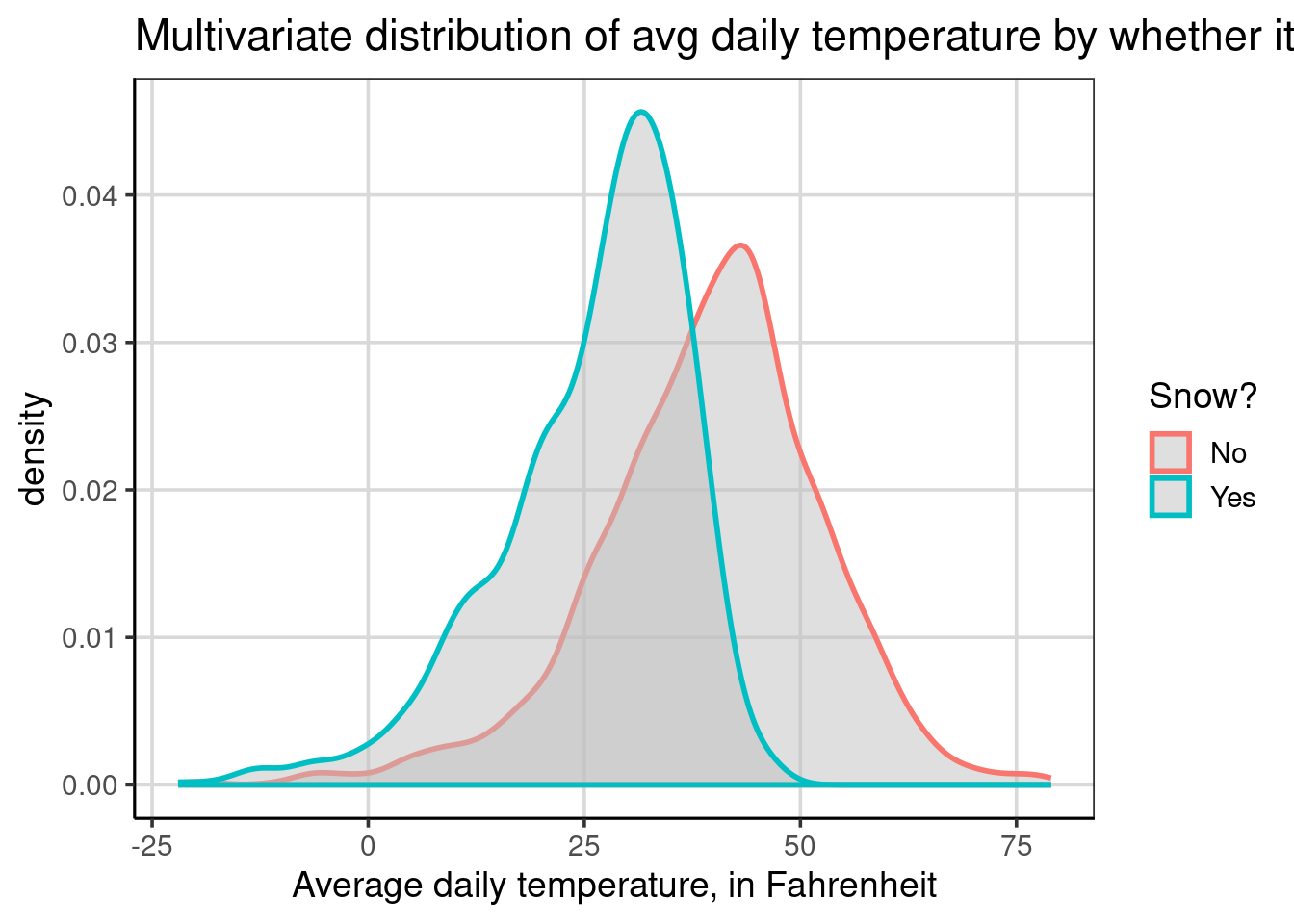This results in a figure that is a bit lighter than the default gray color used in density plots. This lighter gray makes it a bit easier to see the density curves that are plotted behind. When there are more than two groups, this can be an important consideration to take into account to ensure the visualization is easier to interpret. Take the example below which instead of showing whether it snowed it shows the different locations.

gf_density(~ drybulbtemp_avg, data = us_weather,
color = ~ location, size = 1,
fill = 'gray85') %>%
gf_labs(x = "Average daily temperature, in Fahrenheit",
color = "")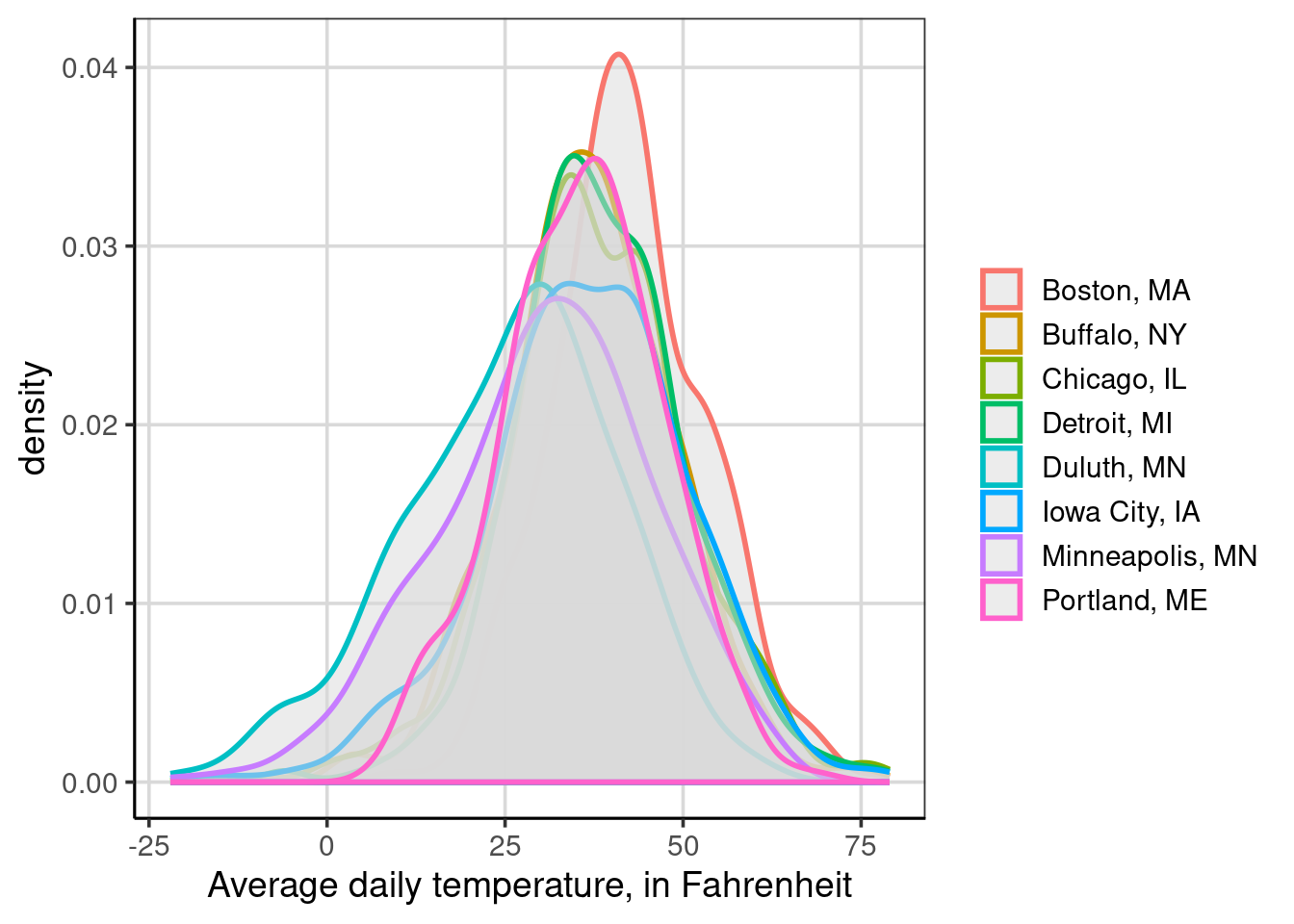Figure 4.1: Multivariate distribution of avg daily temperature by whether it snowed

In this figure, the grayscale was changed from 75 to 85 as well, but you’ll notice that it is still difficult to view all of the locations. This is made more difficult here due to the locations having similar distributional centers. The next section will show an alternative visualization that can help when density curves are difficult to interpret.

### 4.1.3 Violin Plots

Violin plots are another way to make comparisons of distributions across groups. Violin plots are also easier to interpret when there are more than two groups on a single graph. Violin plots are density plots that are mirrored to be fully enclosed. Procedurally, the density curve that was shown above is mirrored or flipped across the x-axis. Let’s explore an example that creates a violin plot with the gf_violin() function and a two-sided formula, drybulbtemp_avg ~ snow which will visualize the average daily temperature by whether it has snowed or not.

gf_violin(drybulbtemp_avg ~ snow, data = us_weather) %>%
gf_labs(y = "Average daily temperature, in Fahrenheit",
title = 'Multivariate distribution of avg daily temperature by whether it snowed',
x = "Snow?")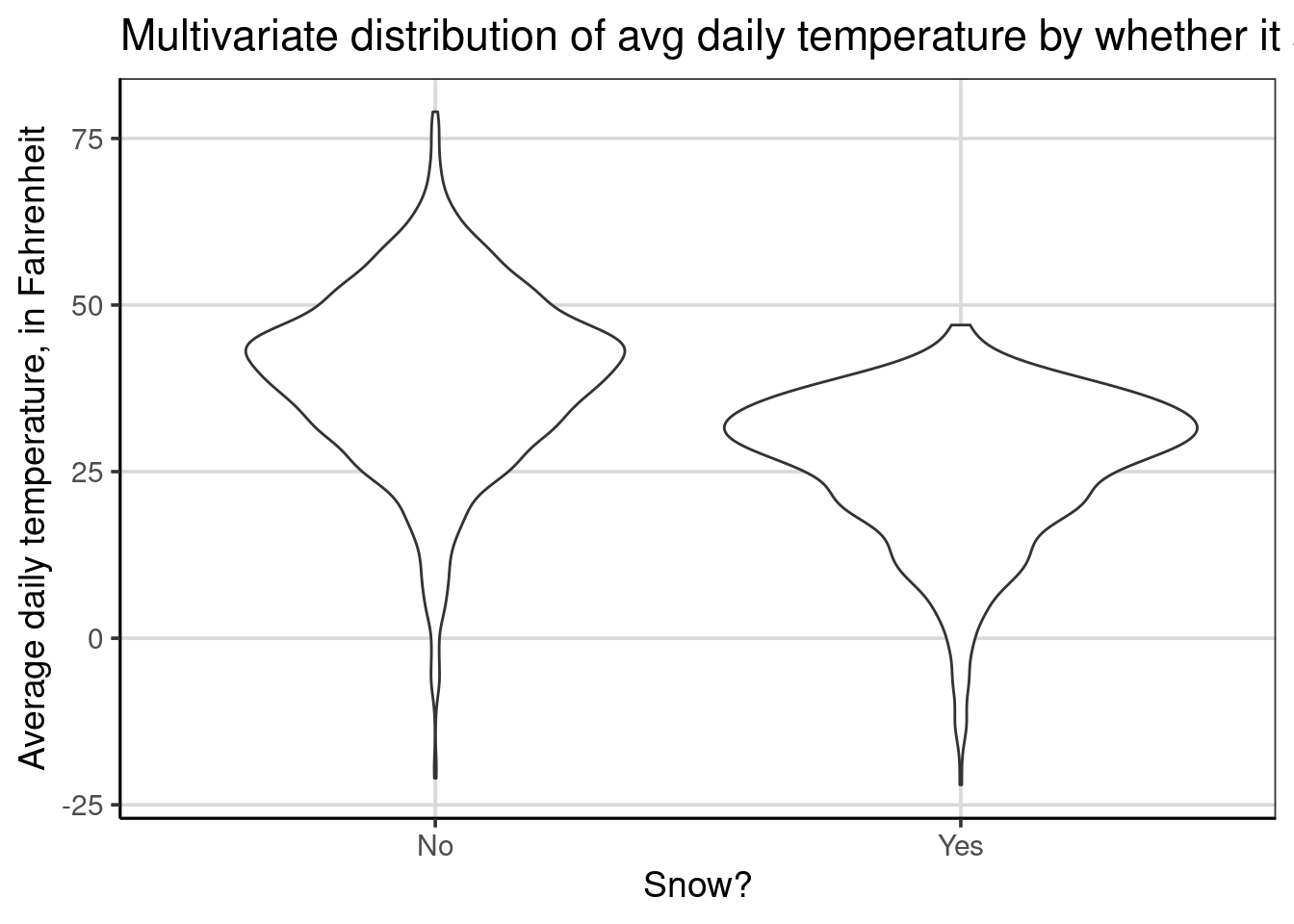By default, the violin plots are oriented vertically, where the temperature is on the y-axis and whether it snowed or not is on the x-axis. This is opposite of what we have been using with histograms and density curves. Fortunately, we can change this behavior by adding a single line of code, gf_refine(coord_flip()). This command flips the x- and y- axes to place the average daily temperature on the x-axis. Throughout the rest of the book, any violin plots shown will have the default axes flipped for consistency with the histograms and density plots.

gf_violin(drybulbtemp_avg ~ snow, data = us_weather) %>%
gf_labs(y = "Average daily temperature, in Fahrenheit",
title = 'Multivariate distribution of avg daily temperature by whether it snowed',
x = "Snow?") %>%
gf_refine(coord_flip())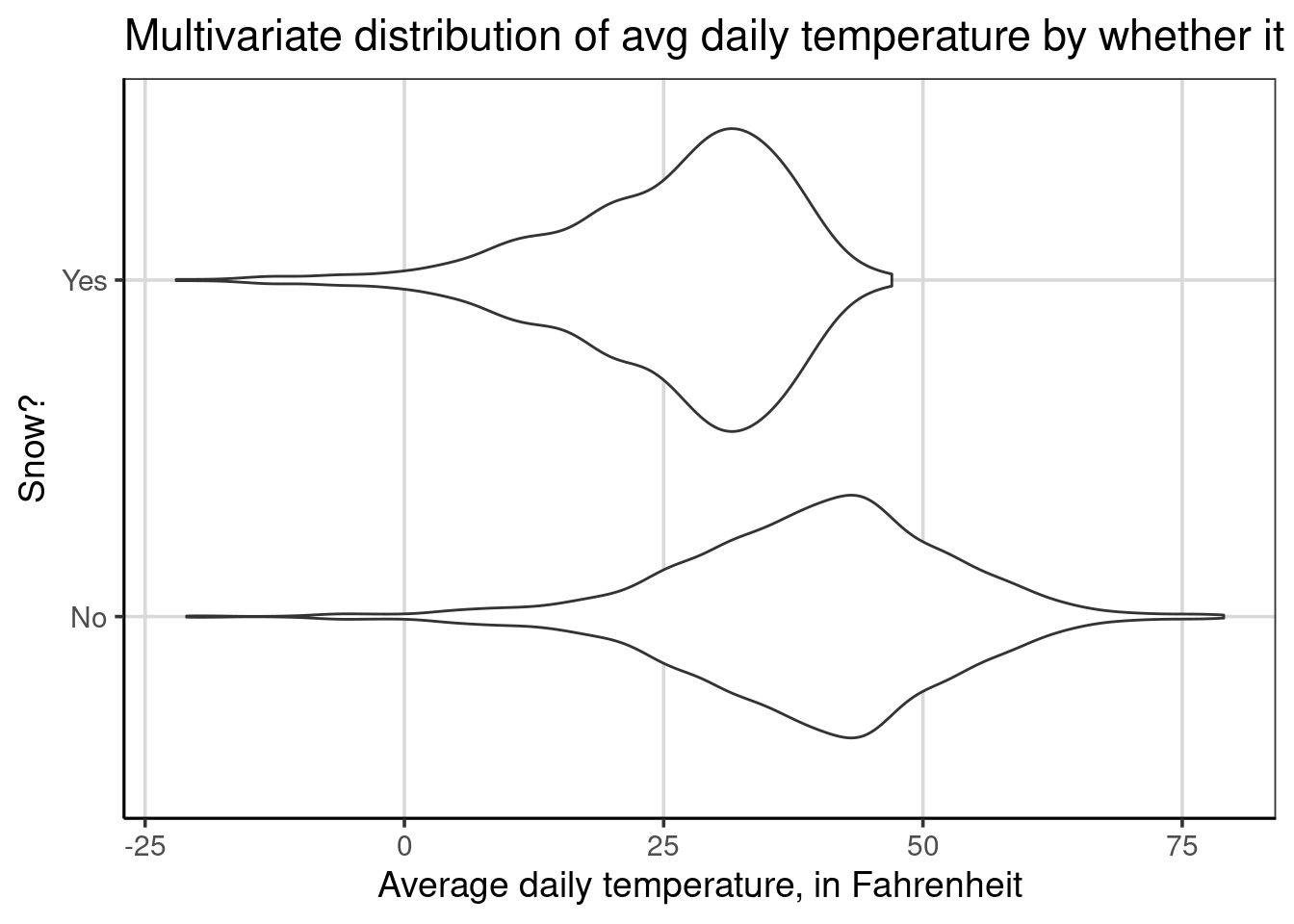Violin plots are depicted for each group separately. This means that there will be the same number of violin plots as the number of groups that are in the attribute on the right hand side of the equation specified inside gf_violin(). In the example above, there is a violin plot for days it snowed and second one for days it did not snow and these violin plots are shown separately and never stacked on top of one another. This is the feature that makes these figures able to handle a larger number of groups more efficiently compared to density of histograms.

From the figure, similar findings discussed earlier can be articulated. For example, there is a higher center and more variation on days that it did not snow. There is also evidence that the distribution for days that did snow is left-skewed whereas the distribution for days it did not snow is more symmetric.

Aesthetically, these figures are a bit more pleasing to look at if they include a light fill color. This is done similar to the density plots shown above with the fill = argument, specified as fill = 'gray85'.

gf_violin(drybulbtemp_avg ~ snow, data = us_weather, fill = 'gray85') %>%
gf_labs(y = "Average daily temperature, in Fahrenheit",
title = 'Multivariate distribution of avg daily temperature by whether it snowed',
x = "Snow?") %>%
gf_refine(coord_flip())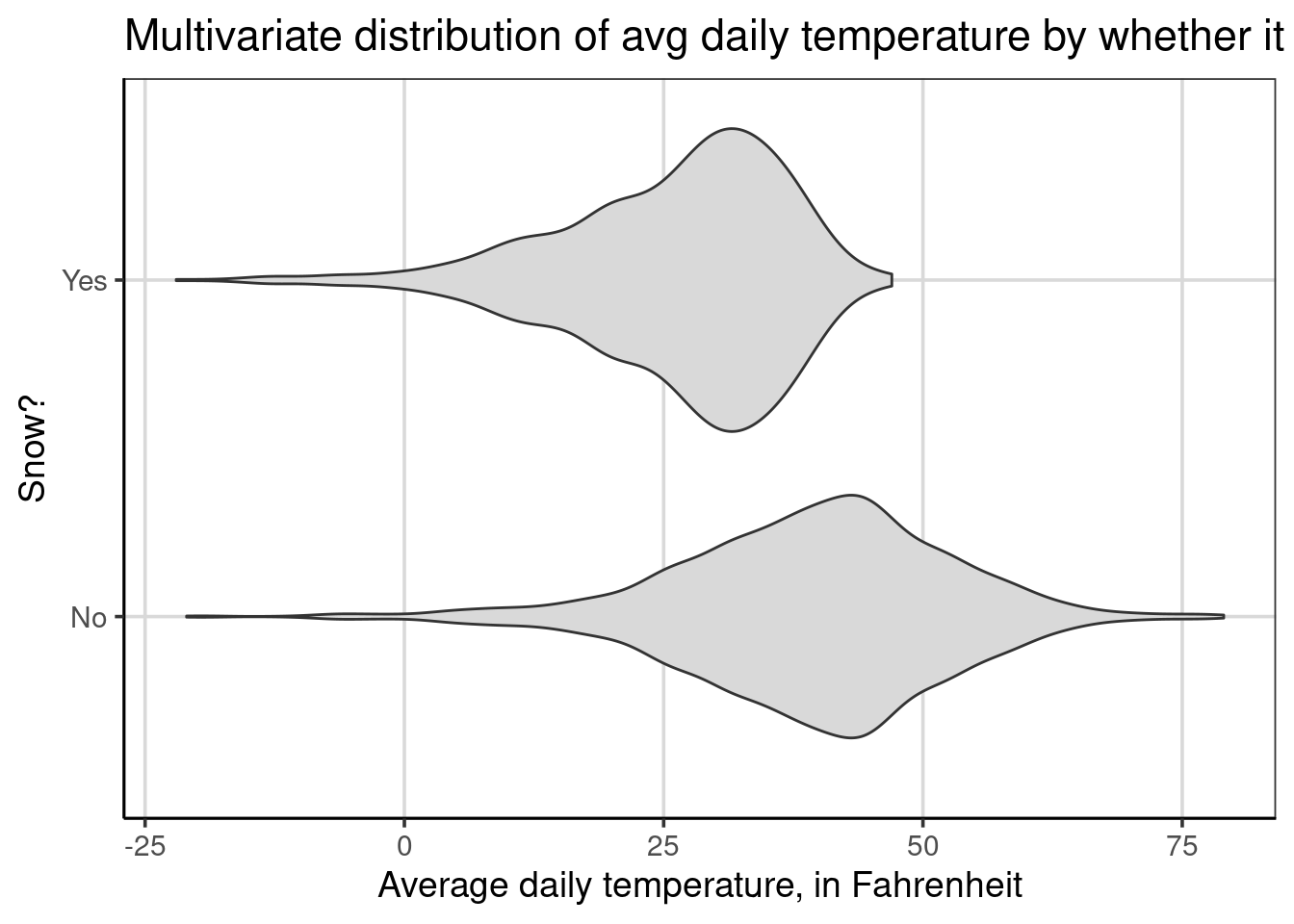Percentiles are another useful feature to aid in the comparison across groups with violin plots. These can be added with the draw_quantiles argument. In the below code, three percentiles are shown, the 10th, 50th, and 90th percentiles are added with the code, draw_quantiles = c(0.1, 0.5, 0.9). Notice that the percentiles are represented as a proportion instead of as a percentage.

gf_violin(drybulbtemp_avg ~ snow, data = us_weather, fill = 'gray85',
draw_quantiles = c(0.1, 0.5, 0.9)) %>%
gf_labs(y = "Average daily temperature, in Fahrenheit",
x = "Snow?") %>%
gf_refine(coord_flip())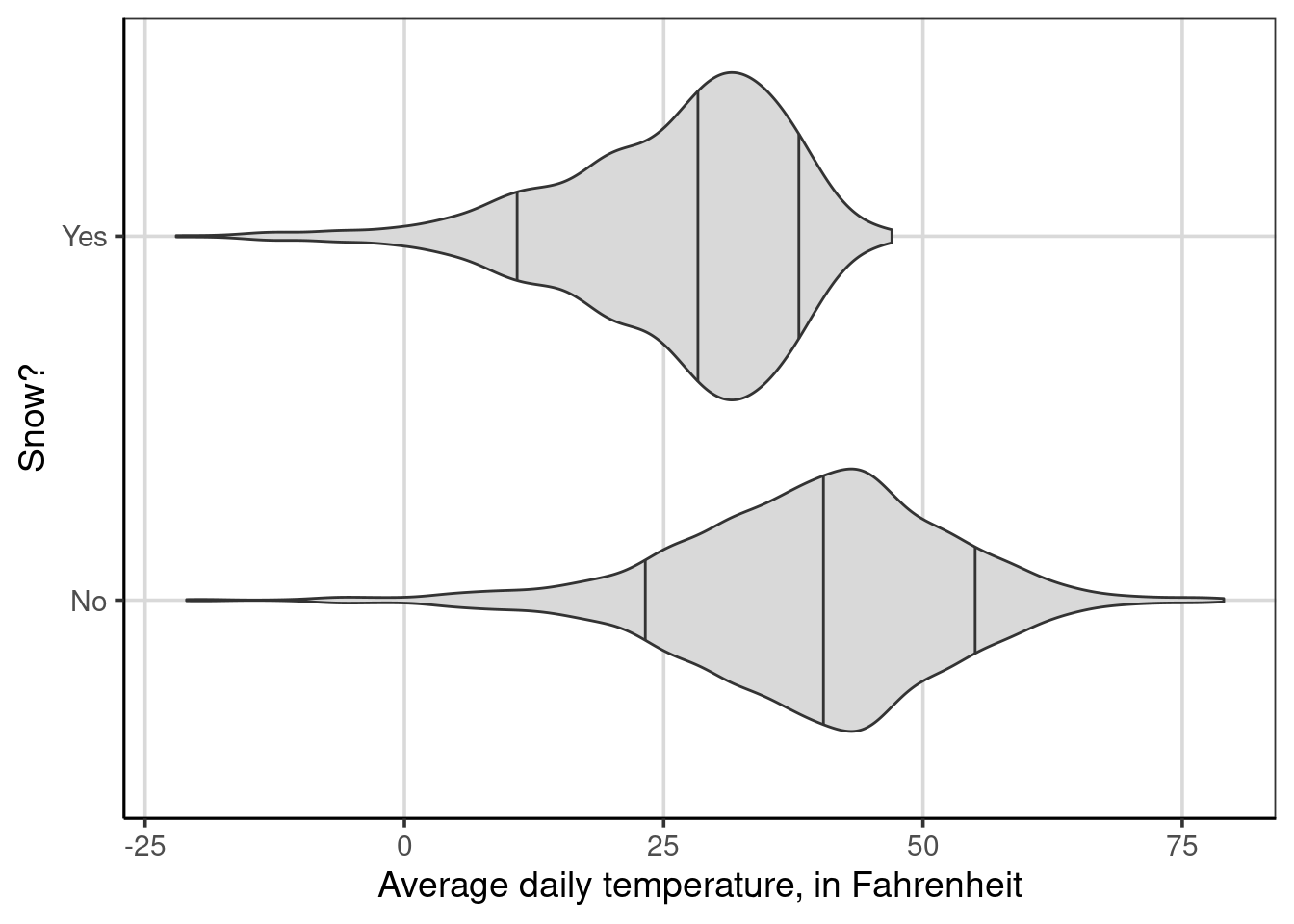Figure 4.2: Multivariate distribution of average daily temperature by whether it snowed

When the percentiles are added, it is now easier to compare the center of the two distributions by using the middle vertical line in each violin plot which represents the 50th percentile or median. In this violin plot, the 50th percentile is lower for days it did snow compared to days it did not snow. Furthermore, the 90th percentile is also lower than the 50th percentile for days that it did snow. This helps to provide evidence that days that it did snow tend to be much colder than typical days, as shown by the 50th percentile, when it did not snow.

Comparing the distance between the 10th and 90th percentiles for each group can also give a sense of the range of typical values. Although this isn’t the interquartile range,17 the difference between the 10th and 90th percentiles reflects a similar quantity. For days that it snowed, the 10th percentile is around 10 to 12 degrees Fahrenheit and the 90th percentile is around 37 to 40 degrees Fahrenheit. Therefore the difference would be about 25 to 30 degrees Fahrenheit. Doing the same process for days it did not snow, the 10th percentile would be around 24 degrees Fahrenheit and the 90th percentile would be around 55 degrees Fahrenheit. The difference would then be around 31 degrees Fahrenheit. This suggests that the spread of the middle 80% of the data in each group are similar, with days in which it snowed showing some evidence of being slightly less spread out over this middle 80%.

#### 4.1.3.1 Violin Plots with many groups

As discussed earlier, visualizing many groups can be done more easily using violin plots compared to density or histograms. This is shown below where the average daily temperature is shown for each of the 8 locations in the US weather data. The 10th, 50th, and 90th percentiles are shown as well for comparison. Take a minute to compare this figure to the one with overlapping density curves in Figure 4.1.

gf_violin(drybulbtemp_avg ~ location, data = us_weather, fill = 'gray85',
draw_quantiles = c(.1, .5, .9)) %>%
gf_labs(y = "Average daily temperature, in Fahrenheit",
x = "Location") %>%
gf_refine(coord_flip())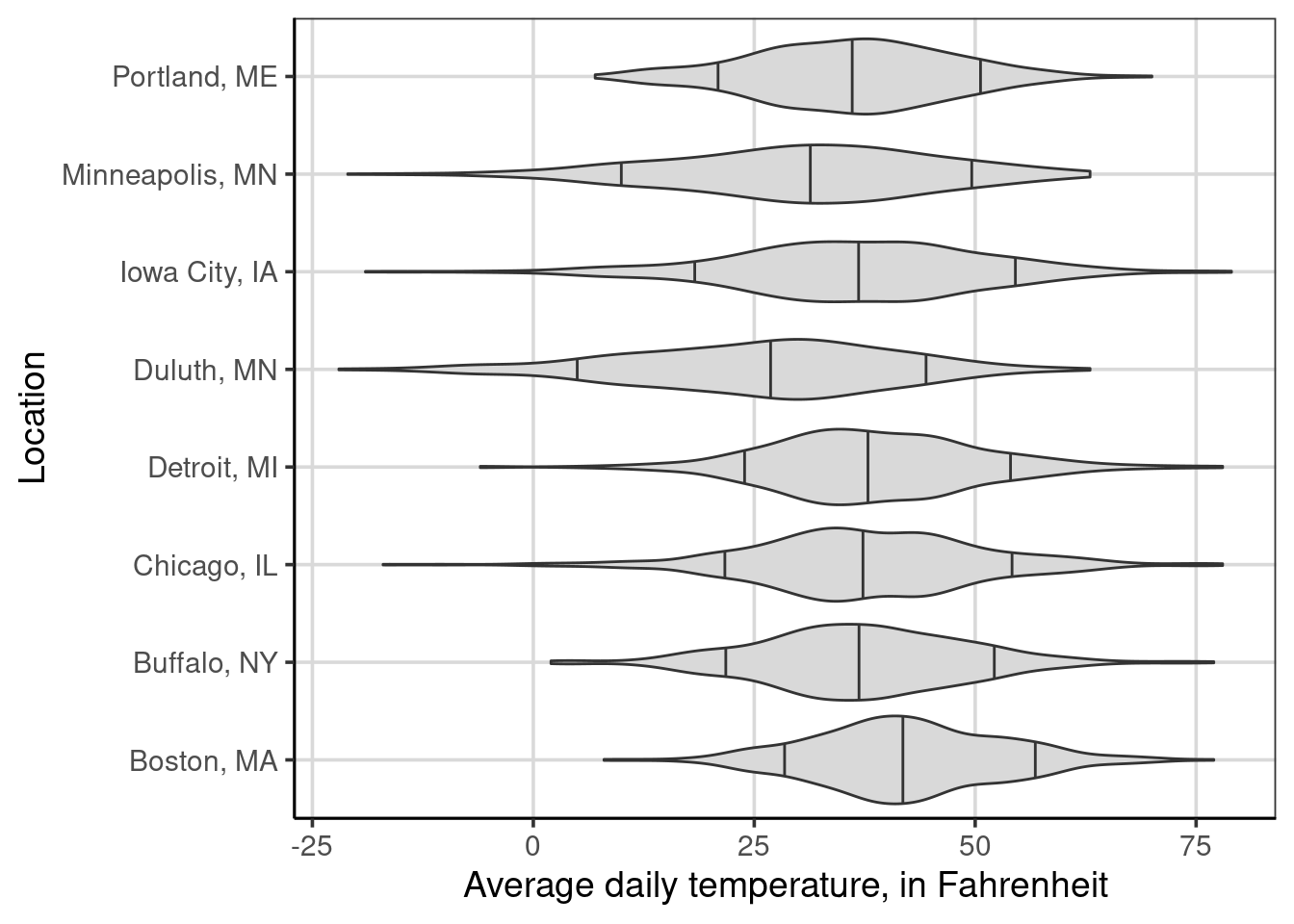Figure 4.3: Average daily temperature by the location of weather measurement.

## 4.2 Faceting

Faceting is a graphical procedure to create multiple figures side by side. This is helpful when there are more than 2 attributes to explore. For example, we have explored the distribution of average daily temperature by whether it snowed or not and also the location separately. Perhaps, there are differences in the distribution of average daily temperatures for whether it snowed or not based on the location. This multivariate visualization now considers two attributes that may help to understand why the average daily temperature may differ.

Below is an example of adding the faceting to a violin plot. The violin plot will explore the average daily temperature by the location. This figure was explored initially in Figure 4.3. This new figure (Figure 4.4) adds one line of code to do the faceting, gf_facet_wrap(~ snow). You may notice that inside the gf_facet_wrap() function, a similar formula notation used by other plotting functions is used. Within this function, a one-sided formula is used where the attribute name is specified after the ~.

gf_violin(drybulbtemp_avg ~ location, data = us_weather, fill = 'gray85',
draw_quantiles = c(.1, .5, .9)) %>%
gf_labs(y = "Average daily temperature, in Fahrenheit",
x = "Location") %>%
gf_refine(coord_flip()) %>%
gf_facet_wrap(~ snow)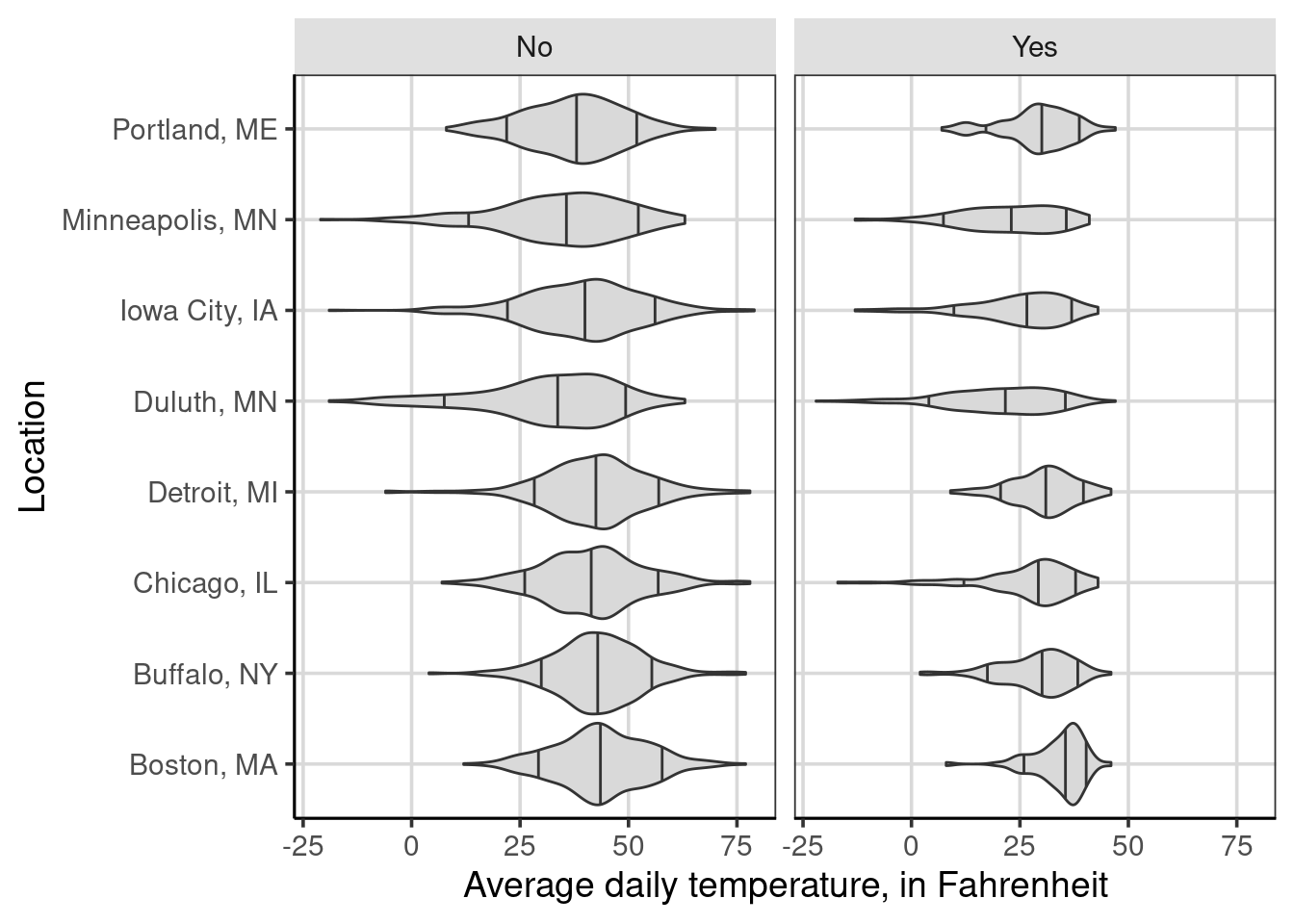Figure 4.4: Average daily temperature by the location of weather measurement and whether it snowed on a given day.

From the figure, the primary difference between Figure 4.3 is the creation of two side-by-side plots, one representing observations when it did not snow (left-side plot) and the other representing observations when it did snow that day (right-side plot). If the two figures were combined, the same figure as in Figure 4.3 happen. The faceting is splitting the observations based on this new attribute, therefore each panel of the figure has less data compared to the overall that did not split the figure by whether it snowed or not.

There are a few differences shown in the figure, primarily related to variation differences. For instance, the variation of average daily temperatures is much smaller for Detroit when it snows compared to when it does not snow. These variation differences are not as pronounced for a city like Duluth or Minneapolis. Similar to previous discussions, there does not seem to be large differences in average daily temperature across the locations.

As a contrast, one instance that may have a larger impact than location, would be month of the year. Below is a faceted figure that instead of location, adds month of year.

gf_violin(drybulbtemp_avg ~ month, data = us_weather, fill = 'gray85',
draw_quantiles = c(.1, .5, .9)) %>%
gf_labs(y = "Average daily temperature, in Fahrenheit",
x = "Month") %>%
gf_refine(coord_flip()) %>%
gf_facet_wrap(~ snow)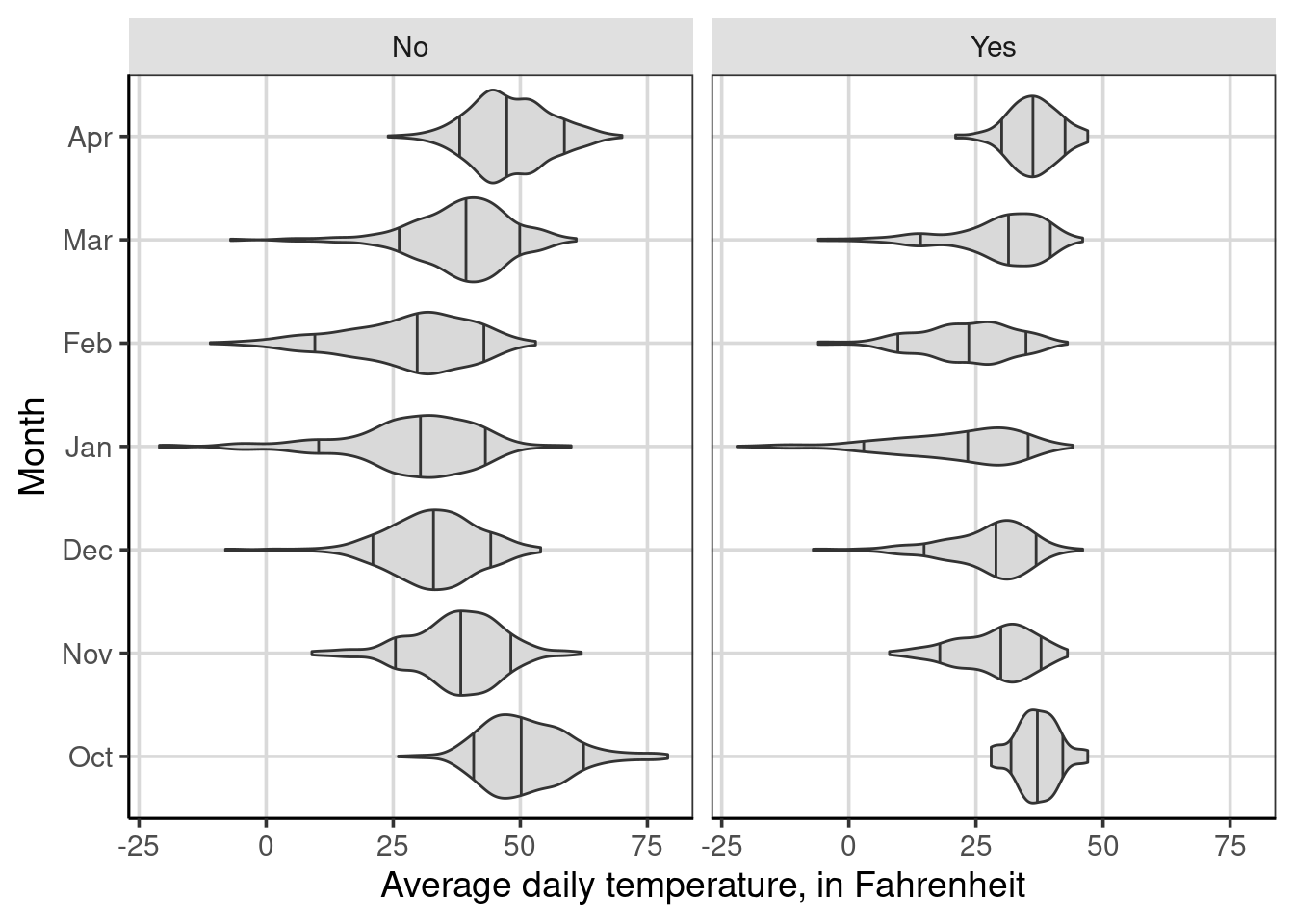Figure 4.5: Average daily temperature by the month of weather measurement and whether it snowed on a given day.

## 4.3 Multivariate Descriptive Statistics

We’ve spent a lot of time trying to reason about other attributes that may be important in explaining variation in our attribute of interest. We started this chapter by exploring visually other attributes that help to understand or explain variation in the primary outcome. For example, we visually explored attributes that may help us understand why average daily temperatures may differ. We found that if it snows on a given day has an impact and month has an impact, but location does not seem to have as large of an effect.

In this section, we will revisit descriptive statistics, or single number summaries of key elements of a distribution. The idea of descriptive statistics will now be generalized to be multivariate in nature. Practically, this means that we will no longer use a single numeric quantity as was done in Chapter 3, instead, we will consider multiple numeric quantities for a single distribution. More accurately, we are now calculating conditional descriptive statistics for conditional distributions. For example, if you look back at Figure 4.5, we conditioned the distribution on month and whether it snowed or not. The conditioning means that the distribution of the average daily temperature was split into separate distributions for each unique combination of the month and snow attributes. In the case of descriptive statistics, we will now compute single number summaries for those conditional or separate distributions. Let’s jump in with a concrete example that computes the multivariate (conditional) descriptive statistics that we explored in Figure 4.2.

Recall from Chapter 3, to compute descriptive statistics the df_stats() function is used. The primary arguments to this function are a formula indicating the attributes to consider followed by the statistics to be calculated. In Chapter 3, the formula used for the df_stats() function were all one-sided as these were univariate descriptive statistics. The formula when calculating multivariate (conditional) descriptive statistics will be two-sided of the following form primary-attribute ~ conditional-attributes. In this formula specification, the primary attribute would represent the attribute the descriptive statistics will be computed for or the attribute of interest. The conditional attribute(s) represent the attribute that the data will be conditioned or split on. Looking at the next code chunk, notice that the formula specification is drybulbtemp_avg ~ snow which means that the descriptive statistics will be computed for the average daily temperature or the primary attribute and this computation will be done for each unique value of the snow attribute. As we know, the snow attribute takes on two unique values, either it snowed or it did not snow. Therefore, the resulting output will contain two rows, one for days in which it did snow and one for when it did not snow.

us_weather %>%
df_stats(drybulbtemp_avg ~ snow, median) 
##          response snow median
## 1 drybulbtemp_avg   No     41
## 2 drybulbtemp_avg  Yes     29

Presented above are the conditional medians for the average daily temperature for days in which it snowed (Yes) compared to days it did not snow (No). These are the middle lines shown in Figure 4.2, but now explicitly calculated. The data were split into subgroups and the median was computed for each of those subgroups instead of pooling all observations.

One thing that is useful to add in when computing conditional statistics, is how many data points are in each group. This is particularly useful when the groups are different sizes, which commonly occurs. To do this, we can add another function, length, to the df_stats() function.

us_weather %>%
df_stats(drybulbtemp_avg ~ snow, median, length) 
##          response snow median length
## 1 drybulbtemp_avg   No     41   2392
## 2 drybulbtemp_avg  Yes     29   1008

This adds another columns which represents the number of observations that went into the median calculation for each group and that there are many more days for these locations where it did not snow. The syntax above also shows that you can add additional functions separated by a comma in the df_stats() function and are not limited to a single function. We will take advantage of this feature later on.

What if we thought more than one attribute was important in explaining variation in the outcome? These can also be added to the df_stats() function for additional conditional statistics. The key is to add another attribute to the right-hand side of the formula argument. Each attribute on the right-hand side of the ~ are separated with a + symbol. The following example computes the median and number of observations for the average daily temperature based on the unique values of whether it snowed by each month.

us_weather %>%
df_stats(drybulbtemp_avg ~ snow + month, median, length) 
##           response snow month median length
## 1  drybulbtemp_avg   No   Oct   50.0    466
## 2  drybulbtemp_avg  Yes   Oct   37.0     30
## 3  drybulbtemp_avg   No   Nov   38.0    309
## 4  drybulbtemp_avg  Yes   Nov   30.0    171
## 5  drybulbtemp_avg   No   Dec   33.0    335
## 6  drybulbtemp_avg  Yes   Dec   29.0    161
## 7  drybulbtemp_avg   No   Jan   31.0    238
## 8  drybulbtemp_avg  Yes   Jan   24.0    258
## 9  drybulbtemp_avg   No   Feb   30.0    256
## 10 drybulbtemp_avg  Yes   Feb   23.5    200
## 11 drybulbtemp_avg   No   Mar   40.0    381
## 12 drybulbtemp_avg  Yes   Mar   32.0    115
## 13 drybulbtemp_avg   No   Apr   47.0    407
## 14 drybulbtemp_avg  Yes   Apr   36.0     73

From the output, there are 14 rows which coincide with the number of months times 2. The 2 here represents the number of categories for the snow attribute, being if it snowed or not. In this case each month by snow combination has data, but if a combination of the attributes on the right-hand side of the equation do not have any data, those rows would not show up in the descriptive statistic summary table. These multivariate descriptive statistics coincide with Figure 4.5, specifically the middle line in these violin plots.

### 4.3.2 Adding more descriptive statistics

Chapter 3 discussed how to add more descriptive statistics to the df_stats() function. This section serves as a reminder for how to do that. In this chapter, we have only computed the median and number of observations, these are useful statistics, but it is often useful to compute descriptive statistics of variation or other location based statistics such as percentiles or means.

To do this, we can continue adding function separated by commas directly at the end of the df_stats() function. For example, we can add the mean and standard deviation to the previous multivariate descriptive statistics tables by adding , mean, sd to the end of the previous df_stats() function.

us_weather %>%
df_stats(drybulbtemp_avg ~ snow + month, median, length, mean, sd) 
##           response snow month median length     mean        sd
## 1  drybulbtemp_avg   No   Oct   50.0    466 51.25751  8.739614
## 2  drybulbtemp_avg  Yes   Oct   37.0     30 37.03333  4.278925
## 3  drybulbtemp_avg   No   Nov   38.0    309 37.52427  9.009927
## 4  drybulbtemp_avg  Yes   Nov   30.0    171 28.77778  7.512952
## 5  drybulbtemp_avg   No   Dec   33.0    335 32.60000  9.318451
## 6  drybulbtemp_avg  Yes   Dec   29.0    161 27.20497  8.892495
## 7  drybulbtemp_avg   No   Jan   31.0    238 28.51681 13.316250
## 8  drybulbtemp_avg  Yes   Jan   24.0    258 20.96899 12.657990
## 9  drybulbtemp_avg   No   Feb   30.0    256 28.01172 12.269676
## 10 drybulbtemp_avg  Yes   Feb   23.5    200 22.74500  9.545447
## 11 drybulbtemp_avg   No   Mar   40.0    381 38.30446 10.005878
## 12 drybulbtemp_avg  Yes   Mar   32.0    115 29.31304  9.812600
## 13 drybulbtemp_avg   No   Apr   47.0    407 47.91646  7.817367
## 14 drybulbtemp_avg  Yes   Apr   36.0     73 36.31507  4.918437

One thing to note when adding more statistics to the df_stats() function is the order with which these show up. The statistics computed show up in the order specified, therefore the statistics from the following command will be median, number of observations (length), mean, and then standard deviation.

1. For more information on why it snows instead of rains, this is an informative description from the National Snow & Ice Data Center↩︎

2. The interquartile range is the difference between the 25th and 75th percentiles↩︎